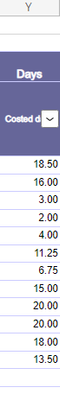cancel
Showing results for
Did you mean:Frequent Visitor

## Summarized values are wrong but grand total is correct

Hello, I have a measure which calculates the FTE (Sum of column25 divide by max value of a Column (Column59) :

FTE = DIVIDE(
SUM('Database (2)'[Column25]),
CALCULATE(MAX('Database (2)'[Column59]), ALL('Database (2)'))
)

I have another measure which calculates all the FTE out of the Top 10 values of FTE :
Outside TOP10_2 = [FTE] - SUMX(
KEEPFILTERS(
TOPN(10, ALL('Database (2)'[Column23]), [FTE])
),
[FTE]
)

(The Column23 is my "Client" Column)
In my case, the FTE measure total is : 266,3 and the sum of the values out of the top10 FTE is : 114,38
In a table, I have two columns, the FTE measure and a column of regional code (Column11). For this case, the summarized values AND the grand total are correct :But, when i replace the FTE measure by the outside_top10 measure : all the summarized values are incorrect (all 0) but the grand total remains correct : 114,38 :Do you know how to solve this ?

5 REPLIES 5Super User

Hi,

Share some data, explain the question and show the expected result.

Regards,
Ashish Mathur
http://www.ashishmathur.comFrequent Visitor

My column 25 is composed of numbers which can be negative and sometimes cells are blank :The column23 is a column with client name. Of course, the same client is present in different lines with different values for the column25 (positive, negative, blank). The interesting thing I noticed is that no matters the other column I'm adding with the measure "Outside TOP2", the summarized values are wrong (either it shows 0 for all values either it shows not all values aggregate). With the measure [FTE], everything works fine (summarized values, you can see the sum of values by regional code is 266) but with the other measure, only the grand total is correctSuper User

@Eliot4 , Try like

Outside TOP10_2 =
Sumx(values('Database (2)'[Column23]),
calculate(
[FTE] - SUMX(
KEEPFILTERS(
TOPN(10, ALL('Database (2)'[Column23]), [FTE])
),
[FTE]
)))Frequent Visitor

Sir I think your formule is close to be the right one but the blank cells in Column25 is is setting the sum equal to 0 each time one line with a regional code has a blank cells in Column25 !Frequent Visitor

The summarized values (i.e FTE outside top 10 by region code) are still incorrect 😣 (all showing 0) but grand total remain correct